# P 1 Chapter 10 Trigonometric Identities Equations jfrosttiffin

• Slides: 28P 1 Chapter 10 : : Trigonometric Identities & Equations [email protected] kingston. sch. uk www. drfrostmaths. com @Dr. Frost. Maths Last modified: 3 rd May 2020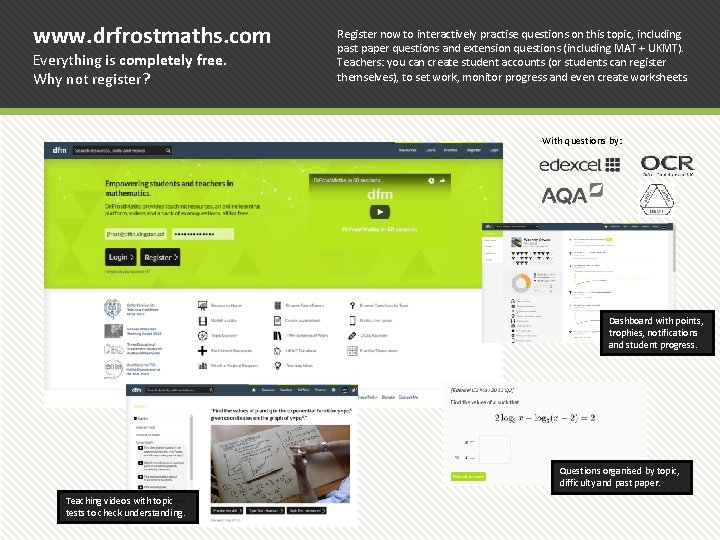www. drfrostmaths. com Everything is completely free. Why not register? Register now to interactively practise questions on this topic, including past paper questions and extension questions (including MAT + UKMT). Teachers: you can create student accounts (or students can register themselves), to set work, monitor progress and even create worksheets. With questions by: Dashboard with points, trophies, notifications and student progress. Questions organised by topic, difficulty and past paper. Teaching videos with topic tests to check understanding.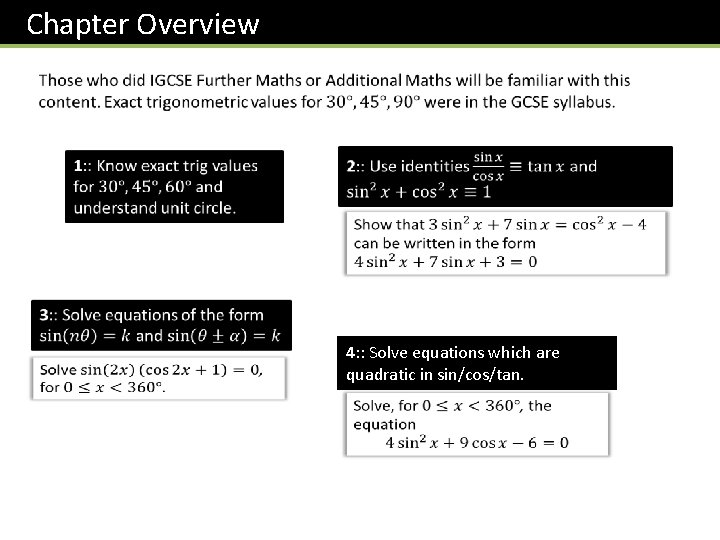Chapter Overview 4: : Solve equations which are quadratic in sin/cos/tan.? Although you will always have a calculator, you need to know how to derive these. All you need to remember: ! Draw half a unit square and half an equilateral triangle of side 2. ? ? ? ? ? ? ?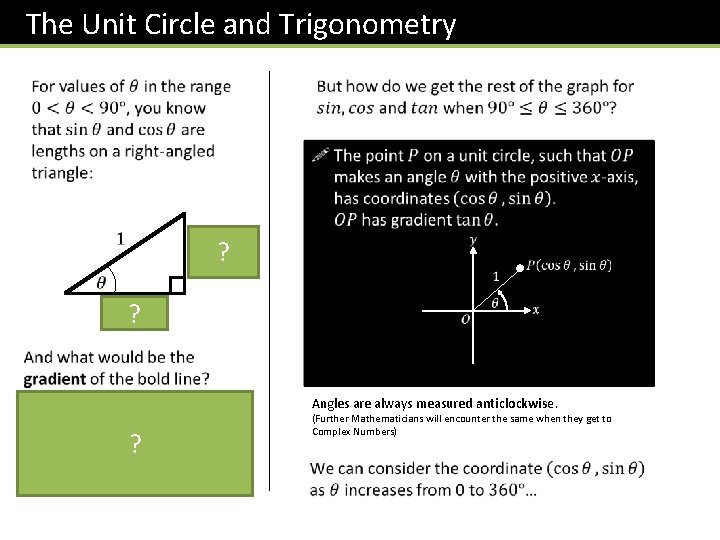The Unit Circle and Trigonometry ? ? Angles are always measured anticlockwise. ? (Further Mathematicians will encounter the same when they get to Complex Numbers)Mini-Exercise ? ? 1 0 0 +ve? +ve 0 1 -ve +ve ? ? Undefined (vertical lines don’t have a well-defined gradient) -ve ? ? -1 ? 0 0 -ve ? +ve 0 ? -1 +ve ? -ve Undefined -ve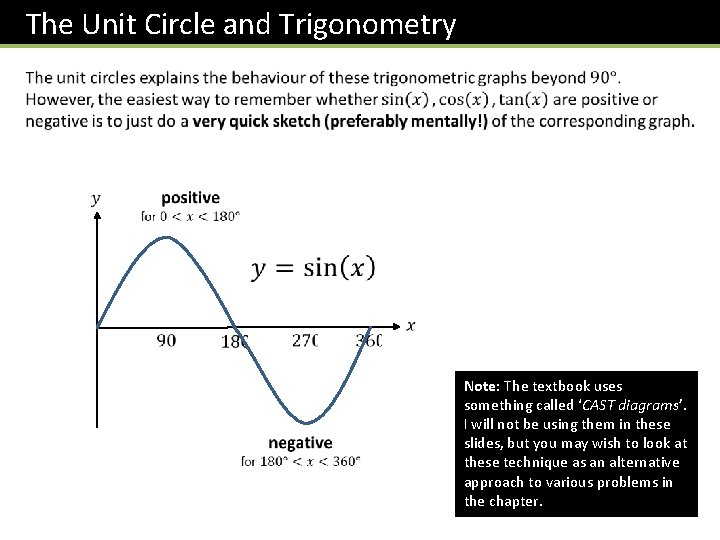The Unit Circle and Trigonometry Note: The textbook uses something called ‘CAST diagrams’. I will not be using them in these slides, but you may wish to look at these technique as an alternative approach to various problems in the chapter.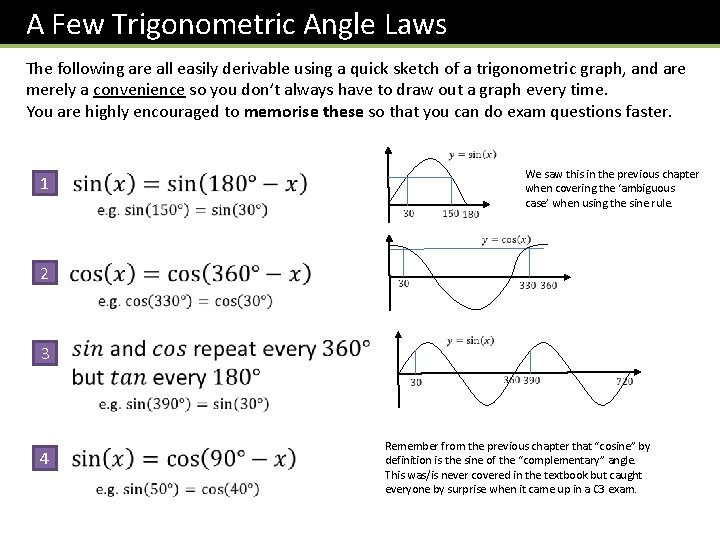A Few Trigonometric Angle Laws The following are all easily derivable using a quick sketch of a trigonometric graph, and are merely a convenience so you don’t always have to draw out a graph every time. You are highly encouraged to memorise these so that you can do exam questions faster. 1 We saw this in the previous chapter when covering the ‘ambiguous case’ when using the sine rule. 2 3 4 Remember from the previous chapter that “cosine” by definition is the sine of the “complementary” angle. This was/is never covered in the textbook but caught everyone by surprise when it came up in a C 3 exam.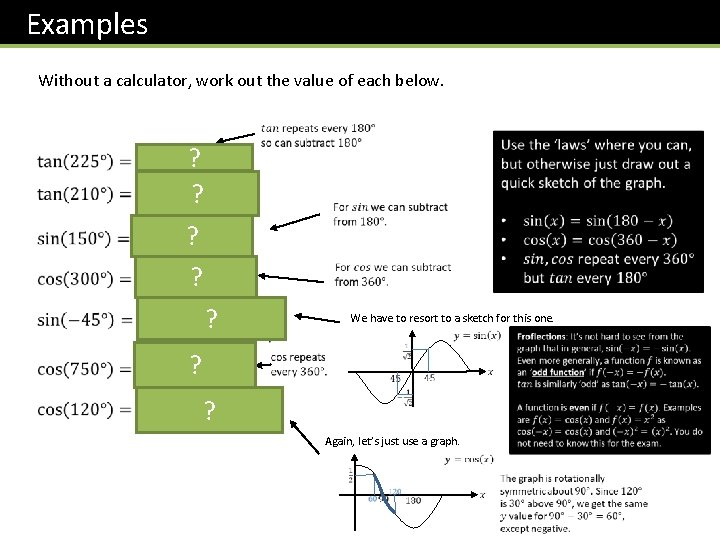Examples Without a calculator, work out the value of each below. ? ? ? We have to resort to a sketch for this one. ? ? Again, let’s just use a graph.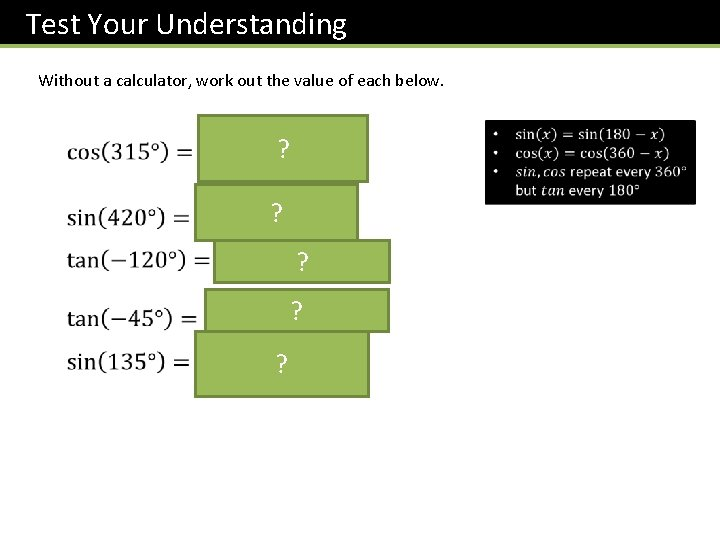Test Your Understanding Without a calculator, work out the value of each below. ? ? ?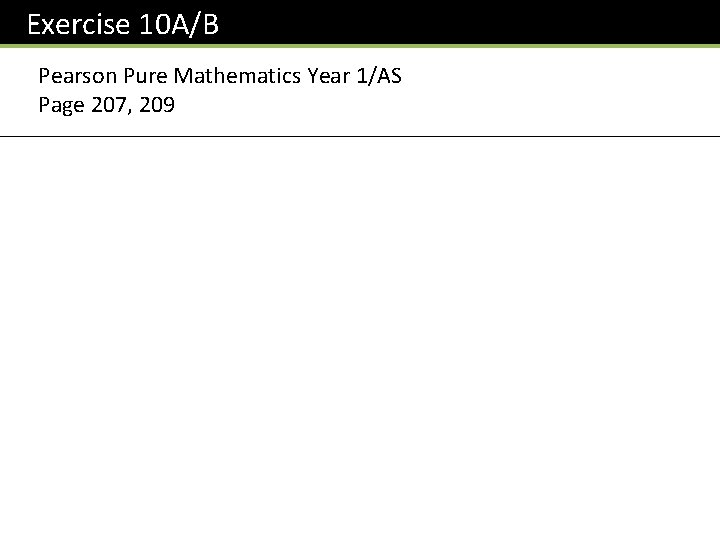Exercise 10 A/B Pearson Pure Mathematics Year 1/AS Page 207, 209Trigonometric Identities Returning to our point on the unit circle… ? ? 1 2 You are really uncool if you get this reference. ? Pythagoras gives you. . . ?Application of identities #1: Proofs ? ? Fro Tip #1: Turn any tan’s into sin’s and cos’s.More Examples Edexcel C 2 June 2012 Paper 1 Q 16 ? ? Fro Tip #2: In any addition/subtraction involving at least one fraction (with trig functions), always combine algebraically into one. ?Test Your Understanding ? ? AQA IGCSE Further Maths Worksheet ?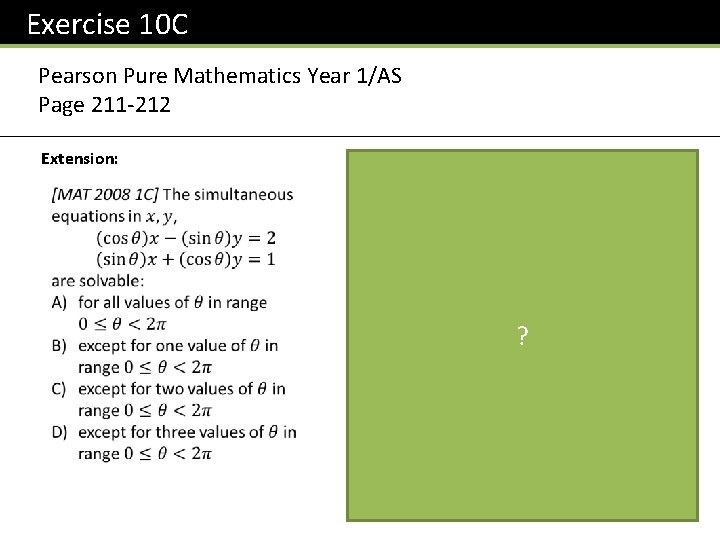Exercise 10 C Pearson Pure Mathematics Year 1/AS Page 211 -212 Extension: ?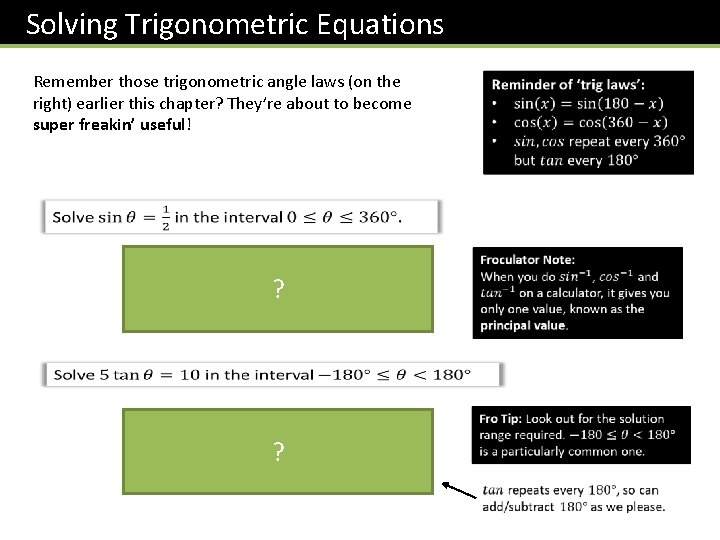Solving Trigonometric Equations Remember those trigonometric angle laws (on the right) earlier this chapter? They’re about to become super freakin’ useful! ? ?Slightly Harder Ones… ? ? Hint: The problem here is that we have two different trig functions. Is there anything we can divide both sides by so we only have one trig function?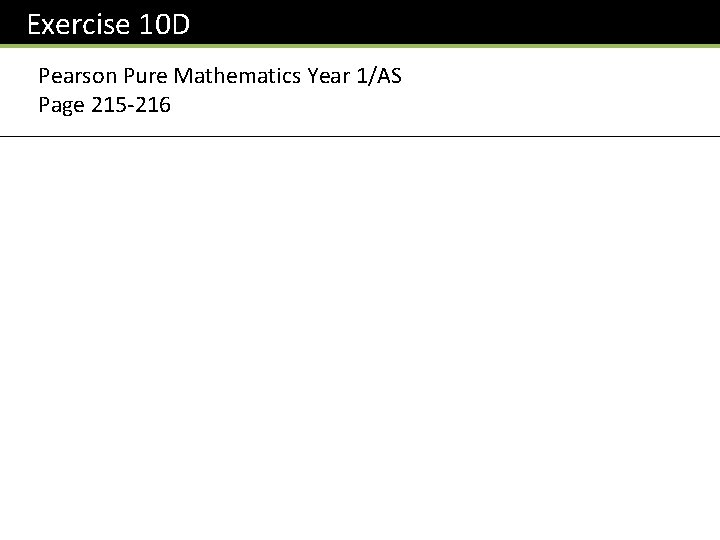Exercise 10 D Pearson Pure Mathematics Year 1/AS Page 215 -216Harder Equations ? STEP 2: Immediately after applying an inverse trig function (and BEFORE dividing by 3!), find all solutions up to the end of the interval. STEP 3: Then do final manipulation to each value.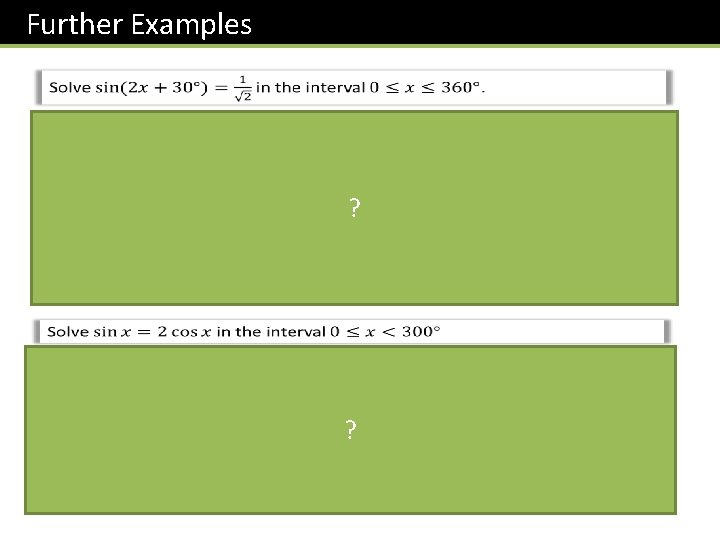Further Examples ? ?Test Your Understanding Edexcel C 2 Jan 2013 Q 4 ?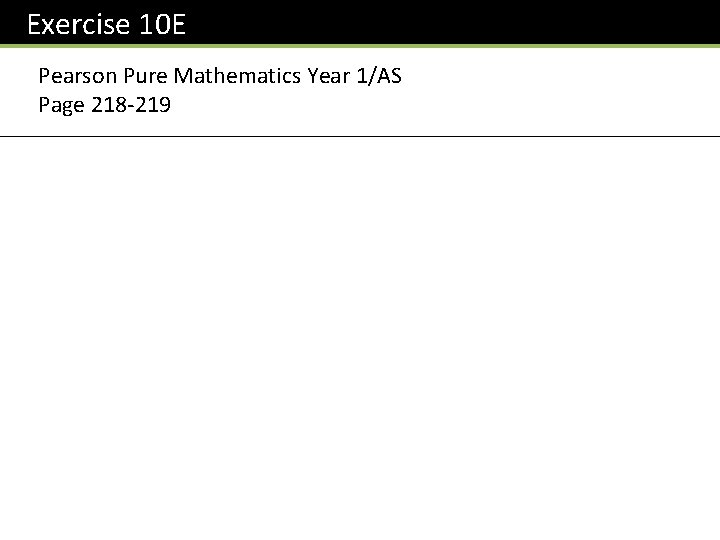Exercise 10 E Pearson Pure Mathematics Year 1/AS Page 218 -219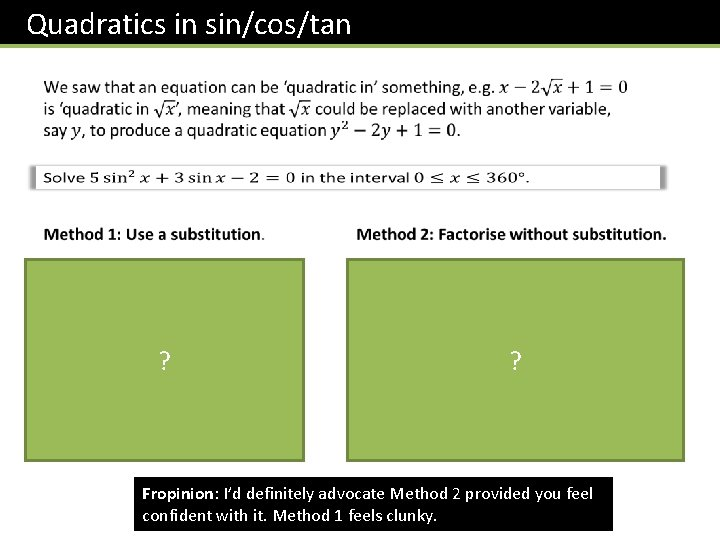Quadratics in sin/cos/tan ? ? Fropinion: I’d definitely advocate Method 2 provided you feel confident with it. Method 1 feels clunky.More Examples Missing the negative case would result in the loss of multiple marks. Beware! ? ?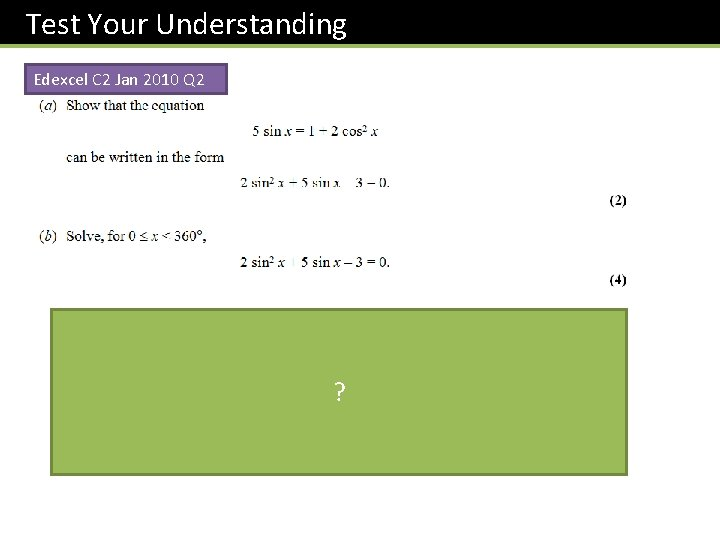Test Your Understanding Edexcel C 2 Jan 2010 Q 2 ?Exercise 10 F Pearson Pure Mathematics Year 1/AS Page 221 -222 Extension 1 2 ? ?